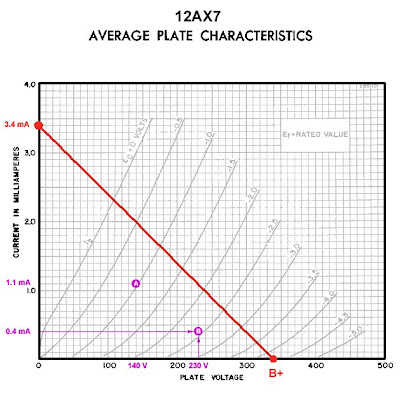## Wednesday, March 4, 2009

### Fender Champion 600 Preamp Bias Part 3

In Part 2a we plotted the maximum current for the first 12AX7 gain stage in a Champion 600. That point was connected to the maximum voltage point to find what's called the load line for the stage.This is the place to come to terms with a essential concept. Every point on the graph of plate curves represents a possible combination of plate voltage and plate current for a 12AX7. Let's choose a few for the sake of illustration. Let's pick Point A at 1.1 milliamps of plate current and 140 volts at the plate. Here's that point graphed onto the 12AX7 plate curves:Now we'll pick another one we'll call Point B at 0.4 millamps of plate current and 230 volts at the plate. Here's that point graphed onto the 12AX7 plate curves:Now we'll a third, Point C, at 1.1 milliamps of plate current and 230 volts at the plate. Here's that point graphed onto the 12AX7 plate curves:Here's the fundamental concept. The load line we've drawn crosses all the possible combinations of voltage and current that can occur in our circuit given the 100K plate load we've chosen - so any dot which plots a combination of current and voltage must lie on this line for it to be a possible occurance in the circuit. We've plotted three different points but only Point C lies on the load line. When the circuit is drawing 1.1 mA of plate current the plate voltage must be the 230 volts indicated by point C NOT the 140 volts indicated by Point A. And with 230 Volts on the plate the circuit must draw 1.1 mA as indicated by Point C, NOT the .4 mA indicated by Point B.

The load line indicates that Point A and Point B do not exist for the circuit given our chosen 100K plate load. Points A and B can still exist as long as we adjust either the current or the voltage so that they lie on the load line:This all may seen somewhat abstract, but it's essential to understanding the biasing of the preamp stage. It should all become clearer as we begin to discuss bias in the next few posts.